# Poker: Finding Expected Values of Low Hands

An error occurred trying to load this video.

Try refreshing the page, or contact customer support.

Coming up next: Lotteries: Finding Expected Values of Games of Chance

### You're on a roll. Keep up the good work!

Replay
Your next lesson will play in 10 seconds
• 0:00 Probability in Poker
• 2:44 Probability of a Full House
• 5:12 Probability of Four of a Kind
• 6:27 Probability of Pairs
• 7:53 Lesson Summary
Save Save

Want to watch this again later?

Timeline
Autoplay
Autoplay
Speed Speed

#### Recommended Courses for You

Lesson Transcript
Instructor: Cathryn Jackson

Cat has taught a variety of subjects, including communications, mathematics, and technology. Cat has a master's degree in education and is currently working on her Ph.D.

In this lesson, you'll learn how to use the combination formula to find the probability of getting some of the lower hands in poker. You'll also test your knowledge by calculating the expected values of additional lower hands in the lesson quiz.

## Probability in Poker

Angel is playing poker with her friends. Each person bets they have the best hand. Angel has a pair of aces. What is the probability that Angel's friends have higher hands? You've probably played cards and wondered the same thing. In this lesson, we'll look at the probabilities of some of the lower hands in poker, like pairs of cards. We will also find the probabilities of slightly higher hands, such as a full house and four-of-a-kind.

We have a formula to help find these probabilities. The combination formula gives us the number of ways you can choose members from a bigger group.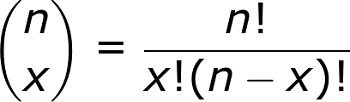The total number of values in the group is n, and x is the number of members we want to choose. We often just call this: n choose x. Each exclamation point represents a factorial, which is a number multiplied by all of the integers below it to 1. For example, 3! means 3*2*1.

Among its many uses, the combination formula allows you to calculate the number of all possible hands in poker. We know that there are 52 cards in a deck (not counting jokers), and that you're dealt five cards. Putting those numbers into the combination formula gives us 52!/(52-5)!5!

Let's see how this formula works. There are 52 possible choices for that first card being dealt, 51 choices for the second card, and so on until we get to the fifth card. That gives us 52*51*50*49*48 possible choices.

Using factorials, we can write this as 52!/47!

However, this number over counts the number of possible poker hands. That's because the order in which your five cards were dealt doesn't change your hand. For example, Angel has a pair of aces and three other cards in her hand no matter what order they were dealt in. Rearranging the order of her cards doesn't change the hand she's been dealt.

How many ways can five cards in a hand be rearranged? There are five ways for the first card, four ways for the second card, and so on, which gives us 5*4*3*2*1, or 5!

We need to divide that first value (52/47!) by 5! so we don't count the same hands as different hands. This gives us 52!/47!5!, the same value you'll get from plugging the numbers into the combination formula.

Now let's discuss the probability of each type of poker hand.

## Probability of a Full House

A full house is a poker hand that includes a three-of-a-kind, or three cards that are all the same value, such as three jacks or three 4s. A full house also includes a pair of cards, such as two aces or two 7s.

First, let's look at all of the possible combinations you can get when dealt five cards. That's a combination of 52 choose 5. Take a look at our combination formula: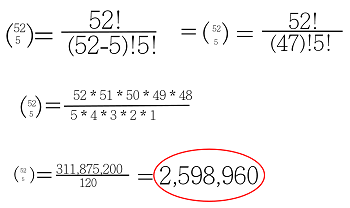Now, let's look at each individual card in this hand and the probability of getting a three-of-a-kind before we discuss the probability of a pair of cards next.

Remember, there are 13 different values of cards in one suit. To get a three of a kind, you must get the same value for each card. Therefore, the number of ways of getting a certain value for each card is 13 choose 1.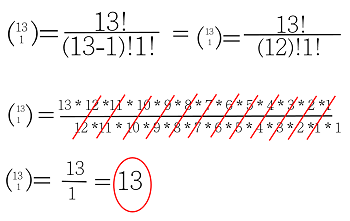We also need to consider that for a three-of-a-kind we will have three out of the four suits. For example, we might have a 7 of spades, a 7 of clubs, and a 7 of diamonds. Therefore there will be 4 choose 3 combinations to consider for the different suits.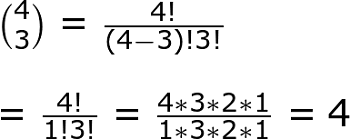By multiplying the combinations for 13 choose 1 and 4 choose 3, we can get our total number of combinations for a three-of-a-kind for this hand. Next, we need to have a pair of cards as our two remaining cards in the full house.

We must consider that we already have three cards in our hand and don't want to get the same value for any of the remaining cards, because that could give us four of a kind. Therefore, the number of possible values for the other two cards would be 12 choose 1, because we have 12 remaining values to choose from. Next, we need to consider the combinations of suits in the deck once more. This time we have 4 choose 2, because there will be only two cards in this pair.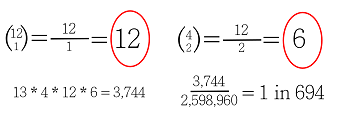Using the numbers we got from all of the different combinations, 13, 4, 12, and 6, we can multiply these combinations together to get a total of 3,744 possible combinations for a full house. Dividing by the total number of hands in the deck, you have approximately a 1 in 694 chance of getting a full house.

That's not a bad probability! Next, let's look at the probability of getting a four-of-a-kind.

To unlock this lesson you must be a Study.com Member.

### Register to view this lesson

Are you a student or a teacher?

#### See for yourself why 30 million people use Study.com

##### Become a Study.com member and start learning now.
Back
What teachers are saying about Study.com

### Earning College Credit

Did you know… We have over 200 college courses that prepare you to earn credit by exam that is accepted by over 1,500 colleges and universities. You can test out of the first two years of college and save thousands off your degree. Anyone can earn credit-by-exam regardless of age or education level.